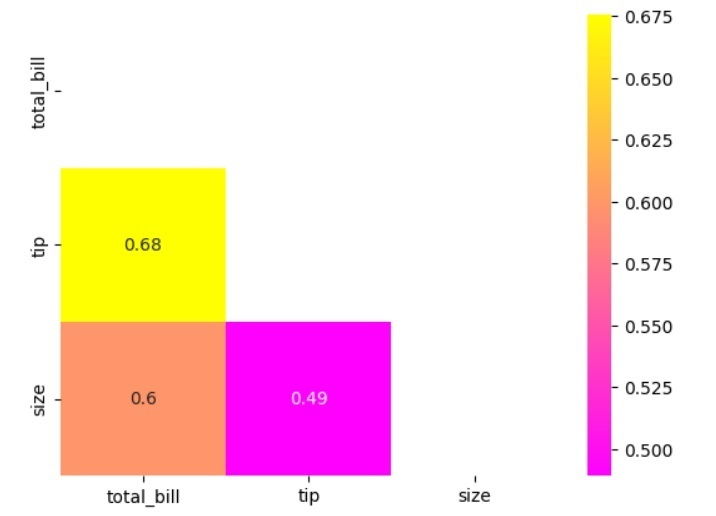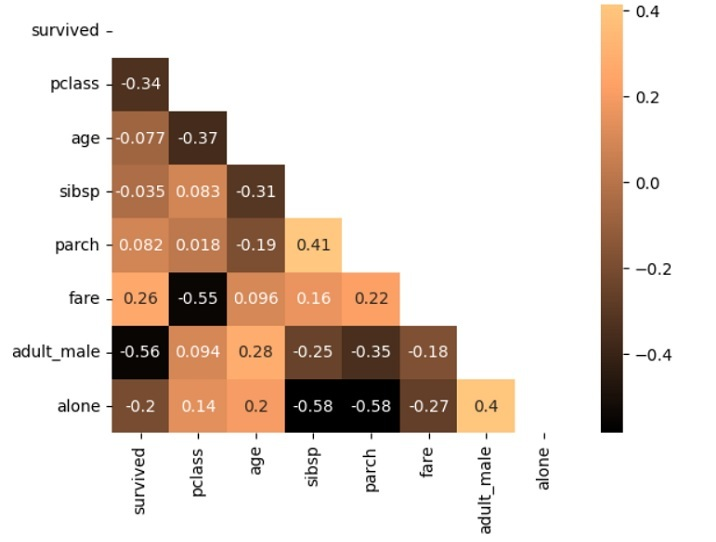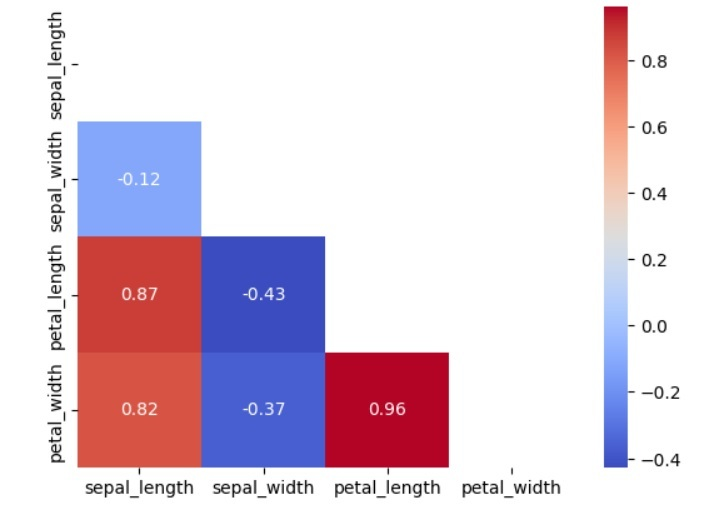# How to create a Triangle Correlation Heatmap in seaborn?

In this tutorial, we will learn to create a Triangle Correlation Heatmap in seaborn; as the name sounds, Correlation is a measure that shows the extent to which variables are related. Correlation heatmaps are a type of plot that represents the relationships between numerical variables. These plots are used to understand which variables are related to each other and the strength of their relationship. Whereas a heatmap is a two-dimensional graphical representation of data using different colors.

Seaborn is a Python library that is used for data visualization. It is useful in making statical graphs. It builds on top of matplotlib and integrates closely with Pandas data structures. It provides several plots to represent the data. With the help of Pandas, we can create attractive plots. In this tutorial, we will illustrate three examples to create Triangle Heatmap. By the end, we will learn the use Seaborn library to create stunning and informative heatmaps.

## Syntax

This is the syntax to create a triangle correlation heatmap.

sns.heatmap(df.corr(),annot=True,fmt=".2f,mask=np.triu(np.ones_like(df.corr(),dtype=bool)))


In this syntax, we created a heatmap using sns.heatmap(). We then pass in the correlation matrix of a DataFrame ‘df’ using ‘df.corr()’. We also set ‘annot=True’ to display the correlation values on the heatmap, ‘fmt=".2f"’ to format the values to 2 decimal places, and ‘mask=np.triu(np.ones_like(df.corr(), dtype=bool))’ to mask the upper triangular part of the heatmap. This makes the heatmap triangular in shape, showing only the lower triangular part that represents the unique correlations.

## Example 1

Here’s an example in which we used ‘tips’ as a dataset. It contains information regarding the tips given to waiters in a restaurant. It includes variables such as the total bill, the size of the party, and the tip amount. Next, we loaded the Tips dataset using Seaborn's ‘load_dataset()’ function and created a correlation matrix using the ‘corr()’ method on the dataset. We then created a Triangle Correlation Heatmap using Seaborn's ‘heatmap()’ function. Finally, we set the attributes and set the color of the map as ‘spring’, and plotted it using the ‘plt.show()’ function. The resulting heatmap shows the correlations between Total Bill, Tip, and Size variables.

import seaborn as sns
import numpy as np
from matplotlib import pyplot as plt
import seaborn as sns
corr = tips.corr()
plt.show()


## Output## Example 2

In this example, we are using the ‘titanic’ dataset, which is a dataset used in machine learning and statistics that contains information about passengers on the Titanic, including their age, sex, ticket class, and whether or not they survived. First, we loaded the Titanic dataset using Seaborn's ‘load_dataset()’ function and created a correlation matrix using the ‘corr()’ method on the dataset. We then create a Triangle Correlation Heatmap using Seaborn's ‘heatmap()’ function and set its attributes. Finally, we displayed it using Matplotlib's ‘show()’ function. The resulting heatmap shows the correlations between the variables Age, Fare, and Class.

import seaborn as sns
import numpy as np
from matplotlib import pyplot as plt
import seaborn as sns
corr = titanic.corr()
plt.show()


## Output## Example 3

In this example, we are using the Iris dataset, another classic dataset used in machine learning and statistics. It contains measurements of the sepal length, sepal width, petal length, and petal width for three species of iris flowers: Setosa, Versicolor, and Virginica. First, we loaded the Iris dataset using ‘Seaborn's load_dataset()’ function and created a correlation matrix using the ‘corr()’ method on the dataset. We then created a Triangle Correlation Heatmap using Seaborn's ‘heatmap()’ function and displayed it using Matplotlib's ‘show()’ function. The resulting heatmap shows the correlations between the variables Sepal Length, Sepal Width, Petal Length, and Petal Width.

import seaborn as sns
import numpy as np
from matplotlib import pyplot as plt
import seaborn as sns
# Create a correlation matrix
corr = iris.corr()
# Create a heatmap using Seaborn
plt.show()


## OutputWe learned that Seaborn is a powerful data visualization library in Python that provides various functions to create different types of visualizations, including heatmaps which are a useful way to visualize the correlations between variables in a dataset, especially when the number of variables is large. Also, Seaborn's ‘heatmap()’ function allows us to customize the color palette and show the correlation coefficients on the heatmap using the cmap and annot arguments, respectively. It also provides several built-in datasets for practising data visualization, such as the Iris dataset, Titanic dataset, and Tips dataset. Creating Heatmaps using Seaborn is very useful for data scientists and analysts who must explore and understand correlations in large datasets. With the help of these heatmaps, data scientists and analysts can gain insights into their data and make informed decisions based on their findings.

Updated on: 11-May-2023

410 Views